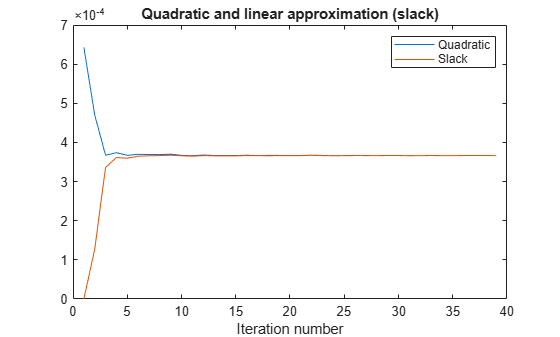# 混合整数二次规划投资组合优化：基于问题

### 问题概要

`$\underset{x}{\mathrm{max}}\left({r}^{T}x-\lambda {x}^{T}Qx\right).$`

`quadprog` 求解器用于求解此二次规划问题。但是，除了简单的二次规划问题之外，您还可能希望以多种方式限制投资组合，例如：

• 投资组合中资产不超过 `M` 种，其中 `M <= N`

• 投资组合中至少有 `m` 种资产，其中 `0 < m <= M`

• 具有半连续约束，意味着对某些固定比例 $fmin>0$$fmax\ge fmin$$x\left(i\right)=0$$fmin\le x\left(i\right)\le fmax$

### 对离散约束建模

$x$ 是资产配置比例的向量，对于每个 $i$$0\le x\left(i\right)\le 1$。要对投资组合中的资产数量进行建模，您需要指示变量 $v$，满足当 $x\left(i\right)=0$$v\left(i\right)=0$，当 $x\left(i\right)>0$$v\left(i\right)=1$。要获得满足此约束的变量，请将 $v$ 向量设置为二元变量，并施加线性约束

`$v\left(i\right)fmin\le x\left(i\right)\le v\left(i\right)fmax.$`

`$m\le \sum _{i}v\left(i\right)\le M.$`

### 目标和连续线性逼近

`$\underset{x}{\mathrm{min}}\lambda {x}^{T}Qx-{r}^{T}x.$`

`${x}^{T}Qx-z={x}_{0}^{T}Q{x}_{0}+2{x}_{0}^{T}Q\delta -z+O\left(|\delta {|}^{2}\right).$`

$x-{x}_{0}$ 取代 $\delta$ 得出

`${x}^{T}Qx-z=-{x}_{0}^{T}Q{x}_{0}+2{x}_{0}^{T}Qx-z+O\left(|x-{x}_{0}{|}^{2}\right).$`

`$-{x}_{k}^{T}Q{x}_{k}+2{x}_{k}^{T}Qx-z\le 0.$`

### MATLAB® 问题表示

• 决定您的变量表示什么

• 表示这些变量中的下界和上界

• 给出线性等式和不等式表达式

```load port5 r = mean_return; Q = Correlation .* (stdDev_return * stdDev_return');```

`N = length(r);`

### 创建问题变量、约束和目标

```xvars = optimvar('xvars',N,1,'LowerBound',0,'UpperBound',1); vvars = optimvar('vvars',N,1,'Type','integer','LowerBound',0,'UpperBound',1); zvar = optimvar('zvar',1,'LowerBound',0);```

`$m\le \sum _{i}v\left(i\right)\le M,$`

`$\sum _{i}v\left(i\right)\le M$`

`$\sum _{i}v\left(i\right)\ge m.$`

```M = 150; m = 100; qpprob = optimproblem('ObjectiveSense','maximize'); qpprob.Constraints.mconstr = sum(vvars) <= M; qpprob.Constraints.mconstr2 = sum(vvars) >= m;```

```fmin = 0.001; fmax = 0.05;```

```qpprob.Constraints.fmaxconstr = xvars <= fmax*vvars; qpprob.Constraints.fminconstr = fmin*vvars <= xvars;```

`qpprob.Constraints.allin = sum(xvars) == 1;`

`lambda = 100;`

`qpprob.Objective = r'*xvars - lambda*zvar;`

### 求解问题

```options = optimoptions(@intlinprog,'Display','off'); % Suppress iterative display [xLinInt,fval,exitFlagInt,output] = solve(qpprob,'options',options);```

```thediff = 1e-4; iter = 1; % iteration counter assets = xLinInt.xvars; truequadratic = assets'*Q*assets; zslack = xLinInt.zvar;```

```history = [truequadratic,zslack]; options = optimoptions(options,'LPOptimalityTolerance',1e-10,'RelativeGapTolerance',1e-8,... 'ConstraintTolerance',1e-9,'IntegerTolerance',1e-6);```

`$-{x}_{k}^{T}Q{x}_{k}+2{x}_{k}^{T}Qx-z\le 0.$`

```while abs((zslack - truequadratic)/truequadratic) > thediff % relative error constr = 2*assets'*Q*xvars - zvar <= assets'*Q*assets; newname = ['iteration',num2str(iter)]; qpprob.Constraints.(newname) = constr; % Solve the problem with the new constraints [xLinInt,fval,exitFlagInt,output] = solve(qpprob,'options',options); assets = (assets+xLinInt.xvars)/2; % Midway from the previous to the current % assets = xLinInt(xvars); % Use the previous line or this one truequadratic = xLinInt.xvars'*Q*xLinInt.xvars; zslack = xLinInt.zvar; history = [history;truequadratic,zslack]; iter = iter + 1; end```

### 检查解和收敛速度

```plot(history) legend('Quadratic','Slack') xlabel('Iteration number') title('Quadratic and linear approximation (slack)')```MILP 解的质量如何？`output` 结构体包含该信息。检查在解处目标的内部计算边界之间的绝对差距。

`disp(output.absolutegap)`
``` 0 ```

```bar(xLinInt.xvars) grid on xlabel('Asset index') ylabel('Proportion of investment') title('Optimal asset allocation')````sum(xLinInt.vvars)`
```ans = 100 ```

```fprintf('The expected return is %g, and the risk-adjusted return is %g.\n',... r'*xLinInt.xvars,fval)```
```The expected return is 0.000595107, and the risk-adjusted return is -0.0360382. ```